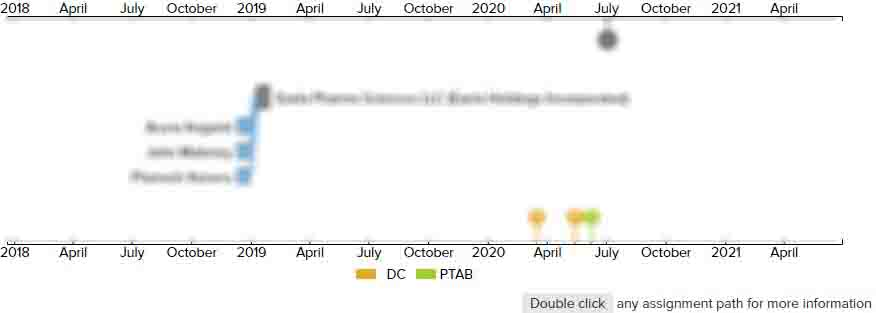# Inverter control device for driving a motor and an air conditioner

• US 7,113,414 B2
• Filed: 03/26/2004
• Issued: 09/26/2006
• Est. Priority Date: 03/28/2003
• Status: Expired due to Fees
##### First Claim
Patent Images

1. An inverter control device for driving a motor, including a rectifying circuit for converting an AC power from an AC power source into a DC power, and an inverter for converting the DC power from the rectifying circuit into an AC power with a desired frequency and a desired voltage to supply the converted AC power into a motor, the rectifying circuit including a diode bridge, and a reactor of a predetermined small capacity connected to the AC input side or DC output side of the diode bridge, the inverter control circuit having a capacitor with a predetermined small capacity for absorbing the regenerative energy of the motor between DC buses of the inverter, the inverter control device comprising:

• a motor voltage command generator that generates a voltage command of each phase of the motor on the basis of a speed command of the motor given from outside;

a voltage detector that detects a DC voltage of the inverter;

a voltage corrector that compares the inverter DC voltage the voltage detector with a predetermined reference value, and calculates a voltage correction coefficient from the result of the comparison,a first motor voltage command corrector that corrects the each phase voltage command, anda second motor voltage command corrector that corrects again the each phase voltage command corrected by the first motor voltage command corrector,wherein the first motor voltage command corrector corrects the each phase voltage command by multiplying the each phase voltage command obtained from the first motor voltage command generator by the voltage correction coefficient produced from the voltage corrector, andthe second motor voltage command corrector corrects again the each phase voltage command once corrected by the first motor voltage command corrector, only when any one of the phase voltage commands corrected by the first motor voltage command corrector is larger than the inverter DC voltage, by multiplying the voltage command of each phase corrected by the first motor voltage command corrector by the inverter DC voltage value, and dividing the product of the multiplication by the maximum value of the phase voltage commands corrected by the first motor voltage command corrector.

• ##### 1 Assignment
Timeline View
Assignment View×
×